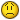# VBA group the number in the Column and Copy to the new worksheet.

#### AmandaM

Hi Everyone,

Currently I have an excel with hundreds of rows, I have to group the rows by the number indicated in column A. For the rows with the same indicator in column A, I would like to copy them into a new worksheet, rename the new worksheet with the indicator.

Sub grouping()
'
'check length of worksheet
Dim i As Integer
i = 1
Range("A1").Select
Do While (Cells(i, 1).Value <> "")
i = i + 1
Loop

i = i - 1

Columns("A:A").ColumnWidth = 10
Range("N:T").EntireColumn.AutoFit

'hide useless columns
Columns("B:M").EntireColumn.Hidden = True

'Consolidation of the worksheet by column A indicator

Dim j As Integer
j = 2
Range("A2").Select

Do

Do While (Cells(j, 1).Value = Cells(j + 1, 1).Value)
j = j + 1
Loop

'Consolidation of the worksheet by column M (which is date) indicator
Dim K As Integer
K = j
Do While (Cells(K, 13).Value = Cells(K - 1, 13).Value)
K = K - 1
Loop

'first row
If j = K And j = 2 Then

With ActiveSheet.XXXXXXXXXX (May I know how can I change the printarea to selection area?, I would like to copy and paste the selection area to new worksheet instead of print it out )
.PrintArea = "\$A\$1:" & "\$T\$" & K
'.LeftMargin = Application.InchesToPoints(0.3)
'.RightMargin = Application.InchesToPoints(0.3)
'.Zoom = False
'.FitToPagesWide = 1
'.FitToPagesTall = False
'.PrintErrors = xlPrintErrorsDisplayed
End With

ActiveWindow.SelectedSheets.PrintOut Copies:=1

Rows(K).EntireRow.Hidden = True

Else

Rows("2:" & K - 1).EntireRow.Hidden = True

With ActiveSheet.PageSetup

.PrintArea = "\$A\$1:" & "\$T\$" & j (Same Issue as above)
'.LeftMargin = Application.InchesToPoints(0.3)
'.RightMargin = Application.InchesToPoints(0.3)
'.Zoom = False
'.FitToPagesWide = 1
'.FitToPagesTall = False
'.PrintErrors = xlPrintErrorsDisplayed
End With

ActiveWindow.SelectedSheets.PrintOut Copies:=1

Rows("2:" & j).EntireRow.Hidden = True

End If

j = j + 1

Loop Until j > i
End Sub